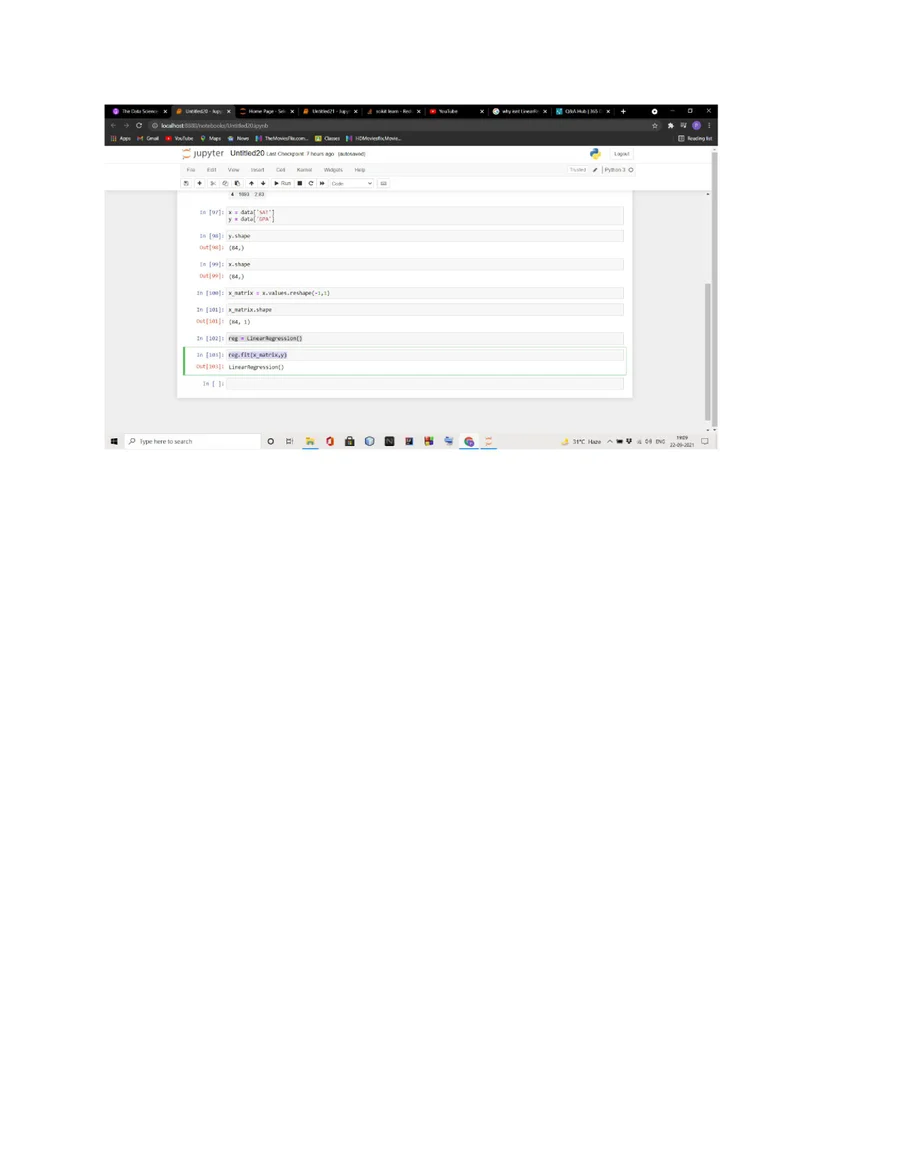Posted on:

22 Sept 2021

0

# linear regression with sklearn

reg = LinearRegression()
reg.fit(x_matrix,y)

expected output was :
LinearRegression(copy_X = True, fit_intercept = True, n_jobs = 1, normalize = False)

real output is:
LinearRegression()

The result occured without any attributes mentioned above. What did I do wrong?